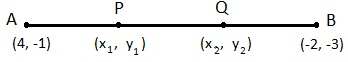Newbie

# Find the coordinates of the points of trisection of the line segment joining (4, -1) and (-2, -3).Q.2

• 0

What is the solution of coordinate geometry of class 10. Please  give me best and easy way to solve exercise 7.2 questions easily   its very important question of this chapter .  Find the coordinates of the points of trisection of the line segment joining (4, -1) and (-2, -3).

Share

1.Let P (x1, y1) and Q (x2, y2) are the points of trisection of the line segment joining the given points i.e., AP = PQ = QB

Therefore, point P divides AB internally in the ratio 1:2.

x= (1×(-2) + 2×4)/3 = (-2 + 8)/3 = 6/3 = 2

y1 = (1×(-3) + 2×(-1))/(1 + 2) = (-3 – 2)/3 = -5/3

Therefore: P (x1, y1) = P(2, -5/3)

Point Q divides AB internally in the ratio 2:1.

x2 = (2×(-2) + 1×4)/(2 + 1) = (-4 + 4)/3 = 0

y2 = (2×(-3) + 1×(-1))/(2 + 1) = (-6 – 1)/3 = -7/3

The coordinates of the point Q is (0, -7/3)

• 0